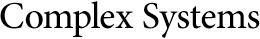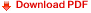Viscosity of a Lattice GasMichel Hénon
C.N.R.S., Observatoire de Nice, France,
B.P. 139, 06003 Nice Cedex, France

Abstract

The shear viscosity of a lattice gas can be derived in the Boltzmann approximation from a straightforward analysis of the numerical algorithm. This computation is presented first in the case of the Frisch-Hasslacher-Pomeau two-dimensional triangular lattice. It is then generalized to a regular lattice of arbitrary dimension, shape, and collision rules with appropriate symmetries. The viscosity is shown to be positive. A practical recipe is given for choosing collision rules so as to minimize the viscosity.